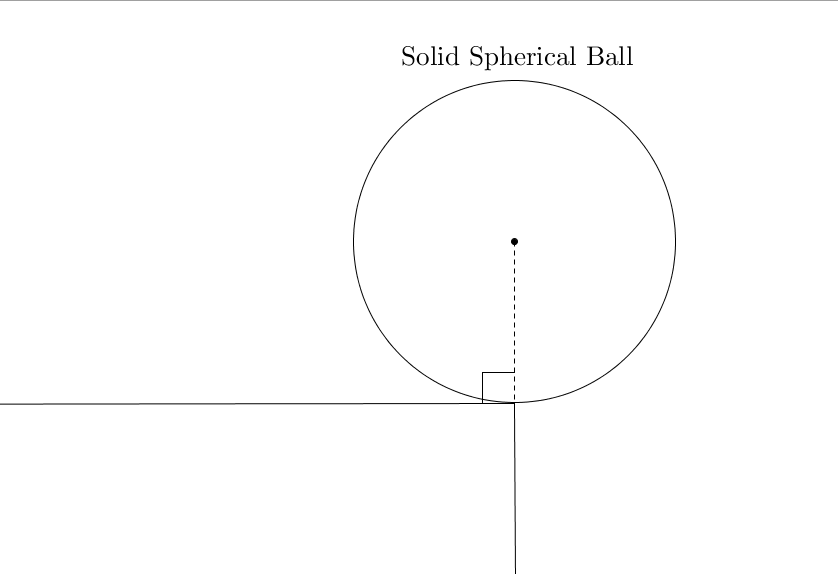# Is it a perfect blend of Mechanics and Calculus?A solid spherical ball is placed carefully on the edge of a table in the position shown in the figure. The coefficient of static friction and kinetic friction between the ball and the edge of the table is 0.5 . It is then given a very slight push. It begins to fall off the table.

Find the angle (in degrees) turned by the ball before it looses contact with the edge.

Details and Assumptions:

1) Find the angle with the vertical.

2) Before solving this try this.

3) You may use WolframAlpha if you want.

×